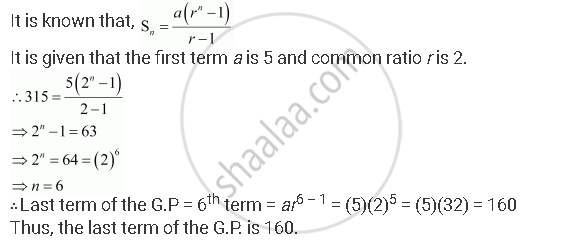# The Sum of Some Terms of G.P. is 315 Whose First Term and the Common Ratio Are 5 and 2, Respectively. Find the Last Term and the Number of Terms. - Mathematics

The sum of some terms of G.P. is 315 whose first term and the common ratio are 5 and 2, respectively. Find the last term and the number of terms.

#### Solution

Let the sum of n terms of the G.P. be 315.Concept: Geometric Progression (G. P.)
Is there an error in this question or solution?
Chapter 9: Sequences and Series - Miscellaneous Exercise [Page 199]

#### APPEARS IN

NCERT Class 11 Mathematics
Chapter 9 Sequences and Series
Miscellaneous Exercise | Q 8 | Page 199

Share